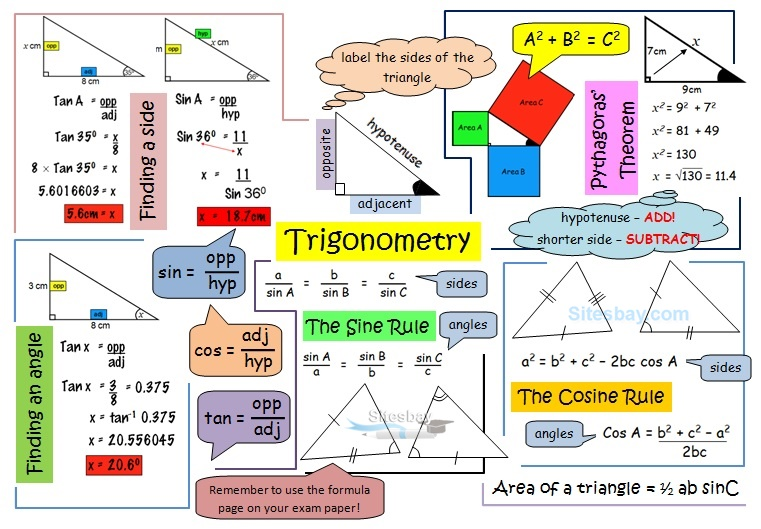# Area Aptitude Problems

## Area Aptitude Tips and Tricks

In this tutorial we discuss about triangle, circle, square and other formula.### Rectangle/Square Formula

• Area of a rectangle = Length x Breadth
• Length of a rectangle = Area / Breadth
• Breadth of a rectangle = Area / Length
• Perimeter of a rectangle = 2(Length + Breadth)
• Area of 4 walls = 2(length+ Breadth) x height
• Area of a Square = (side)2 = 1/2(diagonal)2

### Triangle Formula

• Area of a triangle= (1/2 x Base x Height)
• △ =√{S(S-A) (S-B)(S-C)}, where S=1/2(a+b+c)
• Area of equilateral triangle = √3/4 x a2
• Radius of a in circle of an equilateral triangle of side a = a/2√3
• Radius of a circumcircle of an equilateral triangle of side a =a/√3
• Radius of in circle of a triangle= △/S, Where s=1/2(a+b+c)

### Circle Formula

• Area of a circle= ΠR2
• Circumference = 2ΠR
• Arc length= 2ΠRΘ/360, where Θ is a central angle.
• Area of Sector=1/2(arc length x R) = ΠR2Θ/360
• Area of Semicircle= 1/2ΠR2

### Other shapes Formula

• Area of a parallelogram = (base x height)
• Area of a rhombus= 1/2(product of diagonals)
• Area of a trapezium= 1/2 (sum of parallel sides) x (distance between them)